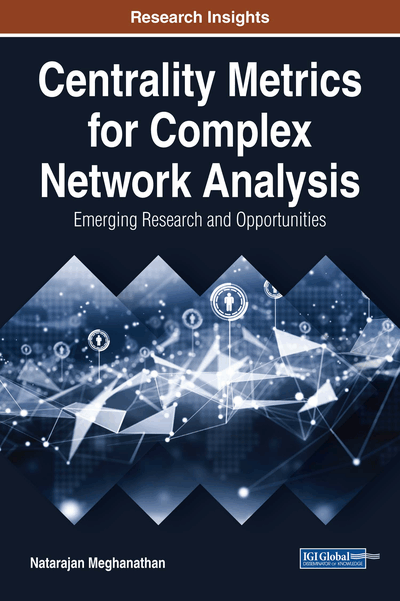# Computationally Light vs. Computationally Heavy Centrality Metrics: Correlation Analysis Between Computationally Light and Computationally Heavy Centrality Metrics

Copyright: © 2018 |Pages: 32
DOI: 10.4018/978-1-5225-3802-8.ch002

## Abstract

In this chapter, the authors analyze the correlation between the computationally light degree centrality (DEG) and local clustering coefficient complement-based degree centrality (LCC'DC) metrics vs. the computationally heavy betweenness centrality (BWC), eigenvector centrality (EVC), and closeness centrality (CLC) metrics. Likewise, they also analyze the correlation between the computationally light complement of neighborhood overlap (NOVER') and the computationally heavy edge betweenness centrality (EBWC) metric. The authors analyze the correlations at three different levels: pair-wise (Kendall's correlation measure), network-wide (Spearman's correlation measure), and linear regression-based prediction (Pearson's correlation measure). With regards to the node centrality metrics, they observe LCC'DC-BWC to be the most strongly correlated at all the three levels of correlation. For the edge centrality metrics, the authors observe EBWC-NOVER' to be strongly correlated with respect to the Spearman's correlation measure, but not with respect to the other two measures.
Chapter Preview
Top

## 1. Introduction

The computation times for the node centrality metrics such as betweenness centrality (BWC) (Freeman, 1977), eigenvector centrality (EVC) (Bonacich, 1987) and closeness centrality (CLC) (Freeman, 1979) as well as for the edge centrality metric such as the edge betweenness centrality (EBWC; Girvan & Newman, 2002) are of Θ(V3) or Θ(VE) time for network graphs of V vertices and E edges. As a result, the computation times for these node and edge centrality metrics are not scalable with increase in the number of nodes and/or edges and we refer to these metrics as computationally-heavy metrics. On the other hand, there exist computationally-light centrality metrics such as degree centrality (DEG) (Newman, 2010) and local clustering coefficient complement-based degree centrality (LCC’DC) (Meghanathan, 2017) at the node-level and the complement of the neighborhood overlap (NOVER’) (Easley & Kleinberg, 2010) metric at the edge level. The focus of this chapter is to conduct extensive correlation analysis of the computationally-heavy node centrality metrics {BWC, EVC, CLC} vs. the computationally-light node centrality metrics {DEG, LCC’DC} as well as the computationally-heavy EBWC vs. the computationally-light NOVER’ at the edge-level.

We conduct the correlation study at three different levels (Triola, 2012): pair-wise ordering (Kendall’s correlation coefficient), network-wide ranking (Spearman’s correlation coefficient) and linear regression-based modeling (Pearson’s correlation coefficient). All the three correlation coefficients are measured in a scale of -1 to 1; (values closer to 1 indicate a stronger positive correlation; values closer to -1 indicate a stronger negative correlation; values closer to 0 indicate no correlation). With Kendall’s concordance-based correlation, we seek to quantify the extent to which a pair-wise ordering between any two vertices (or edges) with respect to a computationally-light metric can be used to predict an ordering of the two vertices (or edges) with respect to a computationally-heavy metric. For example, if LCC’DC(vi) < LCC’DC(vj), we seek to evaluate the extent, we can claim BWC(vi) < BWC(vj)? With Spearman’s rank-based correlation, we seek to quantify the extent we can use a ranking of the vertices (or edges) with respect to a computationally-light metric (like LCC’DC or NOVER’) to rank the vertices (or edges) with respect to a computationally-heavy metric (like BWC or EBWC). With the Pearson’s linear regression-based correlation, we seek to quantify the extent to which we can predict the actual values for a computationally-heavy centrality metric at the node or edge level using the actual values for a computationally-light centrality metric at the node or edge level.

Our research objectives in this chapter are as follows: (i) Identify the computationally-light node centrality metric that is strongly correlated with each of the three computationally-heavy node centrality metrics, for the three different levels of correlations; (ii) Identify the correlation level (pair-wise, network wide-ranking or linear regression-based modeling) for which we would incur the largest value for the correlation coefficient between a computationally-light and a computationally-heavy centrality metric at the node level. (iii) Evaluate the suitability of using NOVER’ as a computationally-light alternative for EBWC for all the three levels of correlation. For correlation analysis involving node centrality metrics, we use a suite of 50 real-world networks (Meghanathan, 2016b) and for correlation analysis involving edge centrality metrics, we use a suite of 47 real-world networks. We omit certain networks for correlation analysis at the edge level due to the computation overhead involved in computing the actual EBWC values.

## Complete Chapter List

Search this Book:
Reset